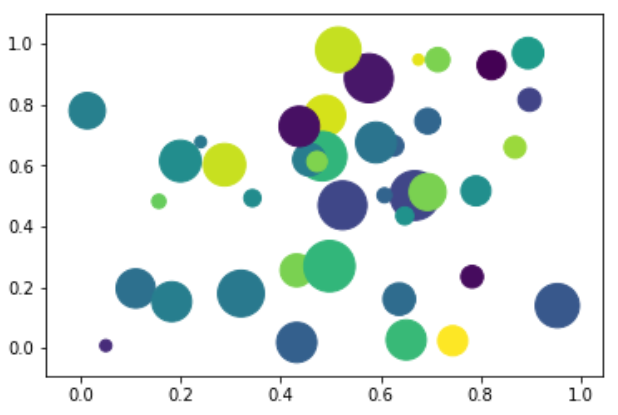# Python – Bubble Charts

Bubble charts display data as a cluster of circles. The required data to create bubble chart needs to have the xy coordinates, size of the bubble and the colour of the bubbles. The colours can be supplied by the
library itself.

## Drawing a Bubble Chart

Bubble chart can be created using the DataFrame.plot.scatter() methods.

```import matplotlib.pyplot as plt
import numpy as np

# create data
x = np.random.rand(40)
y = np.random.rand(40)
z = np.random.rand(40)
colors = np.random.rand(40)
# use the scatter function
plt.scatter(x, y, s=z*1000,c=colors)
plt.show()
```

Its output is as follows −